• matlab怎么生成随机矩阵 Matlab如何生成随机矩阵
千次阅读
2021-04-19 01:43:12

matlab中怎么产生一个随机矩阵？

matlab中通过rand函数产生的是介于0到1(不包括1)之间的伪随机数。更多信息请在matlab命令窗口输入help rand获得帮助信息。用法：1.rand(n)表示产生一个n×n的随机矩阵，n必须是整数，否则会报错。2.rand(m，n)或rand([m n])产生m×n均匀分布的随机矩阵，元素取值在0.0~1.0。3.X=rand(1，10)产生10个0~1的随机数。

怎样用MATLAB按比例生成随机矩阵？

你可以先构造特征值，使得最大特征值除以最小特征值为1000，再随机的生成正交矩阵作为特征向量矩阵，从而重构出所要的矩阵。如下所示，构造了个3*3的矩阵，其条件数为1000：

&gt&gt V = diag([1000 20 1]) % 只要最大除最小等于1000即可

&gt&gt U = orth(randn(3， 3))

&gt&gt A = U*V*U^-1

&gt&gt cond(A)

ans =

1000.0000

下次问编程类问题选择问题分类为编程吧，这样可以贴代码。

请问:怎样用matlab随机生成一个只有0,1元素的矩阵?用什么命令，谢谢啊？

楼上的方法有问题，产生的是0，-1，1的随机矩阵，不是-1和1应该改成：clearclca=randint(3，2)%产生由0和1组成的，m*n维随机矩阵a(find(a==0))=-1%%%%转换成-1和1的矩阵

如何用Matlab生成只含0,1值的随机矩阵,要求限制1的数量？

%选择函数

function A=xuanze(m，n)

A=zeros()

for i=1:m

for j=1:n

A(i，j)=randperm(2，1)-1

end

end

end

%测试函数

m=10

n=5

A=xuanze(m，n)

shu=sum(A(:))%判断1的数量

while(shu&gt=20)

A=xuanze(m，n)

shu=sum(A(:))

end

disp("得到的随机矩阵：")

A

disp(["其中1的个数："])

shu

更多相关内容
• 一种简单快速的生成双随机矩阵的算法。 （矩阵，其中每列和每行的总和正好是 1）。 每个矩阵都是从所有 NxN 双随机的空间中统一选择的矩阵。 注意：生成的矩阵确实是双随机的，但不是证明/检查该算法确实生成了矩阵 ...matlab
• 均匀分布在有限域上所有m×n矩阵集合上的随机矩阵在信息论的许多分支中都起着重要作用。 在本文中，研究了这种随机矩阵的一般化，称为k-good随机矩阵。 结果表明，在最小秩距离min {m，n}-k + 1的最大秩距离（MRD）...研究论文
• MPI程序处理一个随机矩阵乘以一个随机矢量的并行计算 (1)矩阵维度N是可以任意调节；固定一个核数，测试计算时间随N增长的趋势，估计一下N多大时，计算时间需要大约一个月。 (2)统计使用不同核数(1, 2, 3, 4)下计算...mpi
• 本代码主要利用MATLAB工具实现MATLAB——创建标准正态分布随机矩阵，简单明了，易于理解
• 某些众所周知的非Hermitian随机矩阵类别（例如，复杂的Ginibre系综）的特征值PDF可以解释为二维域中一组分等离子体系统的Boltzmann因子。 我们以系统的方式解决此主题，确定非Hermitian高斯随机矩阵G的Ginibre集合的...
• 首先介绍了与无线通信密切相关的随机矩阵理论数学背景;接着阐述了该理论与无线通信系统的紧密联 系,回答了为什么要在无线通信系统中使用随机矩阵理论的问题;最后以运用随机矩阵理论分析大维数系统接收 机性能为例,...
• 这个程序是我参考了网上的一些方案自己总结了比较简单的方法，代码如下： from numpy import random import numpy as np array = random.randint(1,20,size=(10,10)) print(array) np.savetxt('E:\\test.csv',array,...
• 我不知道这是否是第一个，但我很确定你们可以完全理解这个简短而简洁的 matlab 代码可以做什么，即生成任何给定顺序的随机矩阵，随机输入从 {1,-1} 中选择。 事实上，该代码可以扩展为生成任何随机矩阵，其元素是从...matlab
• 我们研究了高斯Unit集合体（GUE）随机矩阵模型中频谱形状因子的后期高原行为。 在平稳状态下，频谱形状因数的时间导数并不严格为零，而是由于1 / N扩展中的非扰动校正而非零。 我们认为这种非摄动校正来自随机矩阵...
• 基于随机矩阵理论的WSN异常节点定位算法.pdf
• This book gives a coherent and detailed description of analytical methods devised to study random matrices. These methods are critical to the understanding of various fields in in mathematics and ...
•研究论文
• 用于生成 (1,-1) 的随机矩阵 A 的更短代码，无循环matlab
• 代码为从一个已知矩阵随机选取n列生成一个包含原数据的子矩阵
• RMT_Theory_Applications 随机矩阵理论的一些重要性质及其在多个领域中的应用
• 1、 利用随机数生成两个4×4的矩阵A和B，前者范围为30~70，后者范围为101~135。 要求： ① 将两个矩阵相加结果放入C矩阵。 ② 将矩阵A转置。 ③ 求C矩阵中元素的最大值和下标。 ④ 以下三角形式显示A，上三角形式...
• 基于伪随机矩阵的LT码编译码算法，赵明华，唐祖平，针对导航电文数据量小的特点，本文提出了基于伪随机矩阵的LT编码方案以及译码性能优于置信度传播-最大似然联合(BPML)译码算法的增强卫星导航
• (1) rand：产生均值为0.5、幅度在0~1之间的伪随机数 (2) randn：产生均值为0、方差为1的高斯白噪声 (3) randperm(n):产生1到n的均匀分布随机序列 (4) normrnd(a,b,c,d)：产生均值为a、方差为b大小为cXd的随机矩阵在...

(1) rand：产生均值为0.5、幅度在0~1之间的伪随机数 (2) randn：产生均值为0、方差为1的高斯白噪声 (3) randperm(n):产生1到n的均匀分布随机序列 (4) normrnd(a,b,c,d)：产生均值为a、方差为b大小为cXd的随机矩阵

在MATLAB中，我们常常会用到一些随机数据，那么这些数据是怎么生成的呢？下面一起来看一看

方法

unifrnd (a,b) ;产生一个[a,b]的均匀随机数

用rand函数生成元素均为0-1之间的随机数的矩阵，然后逐个判断，0.5一下的改为0，0.5以上的改为1： A=rand(m,n); for i=1:m for j=1:n if A(i,j)

rand (m, n)；产生m*n阶［０，1］均匀分布的随机数矩阵

生成n个uniform(0,1)随机数x，然后计算乘积A=xx'，A是一个n*n矩阵。 两个独立随机数的乘积的期望是1/4，(虽然可能不再是uniform分布了)。 那么取G=(A>1/4)，G就会是一个对称的矩阵。 如果需要，对角线上的乘积期望不是1/4，可以特殊处理一下。

binornd(n,p,[M,N,P,...])产生的是服从二项分布的随机数，二项分布的参数为：n和p

删除多余变量矩阵 直接ctrl+s 就能保存当前工作区下的变量矩阵 也有函数save 用于保存 上面第一种方法比较简单 且方便 save函数 语言设置一些参数 具体的参数设置记得不是很清楚了 但也是比较简单的 可以help 一下进行了解 非常简单 不知道你问

unidrnd(N,m,n) ；产生m*n阶离散均匀分布的随机数矩阵；产生一个数值在1-N之间的mm*nn矩阵

在matlab中“建立5*6的随机矩阵，其元素为【100,200】范围内的随机整数”通过random函数来进行实现。 matlab中random函数用法为：y=random('norm',A1,A2,A3,m,n)。式中：A1，A2，A3为分布的参数，m，n用来指定随机数的行和列，name的取值有相关的

exprnd (mu ,m, n)产生m*n阶期望值为mu的指数分布的随机数矩阵

1、首先启动matlab，启动界面如图所示。 2、在命令行窗口中输入代码：[0:0.1:1]。 3、点击回车，可以看到结果从0开始每次增加0.1到1结束。 4、赋予变量x从0到1等值增大的值。在命令行窗口中输入代码：x=[0:0.1:1]。 5、点击回车后便会显示出x变

扩展阅读，以下内容您可能还感兴趣。

怎样用MATLAB生成一个4行5列的间的随机矩阵

rand(4,5) % 四行五列(0,1)之间的随机矩阵

% 如果范围不在0-1之间，可以相应调整

ceil(rand(4,5)*10) % [1,10]之间随机整数

matlab 随机生成每列只有一个1 其余为0的矩阵

m=10;%行数

n=15;%列数

A=zeros(m,n); %生成全零矩阵

b=randi(m,[1 n]); %生成n个从1到m的随机整数

ind=sub2ind([m,n],b,1:n);%形成位置

A(ind)=1;%将对应位置变为1

matlab中怎么随机生成一个M*N矩阵，使每列和为一固定值，每个元素为整数？

% function num=randn(min_n,max_n,m,n)

%思路：

%对于某一列，先产生该列第一个随机数a，其范围为[min_n,max_n]

%然后产生该列第二个随机数b，其范围为[min_n,max_n-a]

%之后产生该列第三个随机数c，其范围为[min_n,max_n-a-b]

%终止条件为max_n-a-b<=min_n

min_n=2;%最小值

max_n=100;%最大值

m=5;%行数，注：行数不宜大，否则0元素过多

n=30;%列数

num=zeros(m,n);%初始化

for i=1:n

for j=1:m

if round(max_n-sum(num(1:j,i)))>min_n%随机数产生的终止条件

num(j,i)=floor(min_n+(max_n-sum(num(1:j,i)))*rand);%产生随机数

end

end

end追问有点问题，就是每列最后一个应为总数减前面总数的差，加一句

num(m,i)=max-sum(num(1:m-1,i));%每列最后元素为剩下的个数

就行了，还是谢谢提供思路。追答嗯 不好意思我遗漏了 难怪会那么多0 嘿嘿

MATLAB 随机产生一个矩阵序列

生成一个元素为0-11之间的M*N维矩阵，这里以M=6，N=9为例，在MATLAB主窗口中输入R=round(11*rand(6,9))，回车。更多追问追答追问要随时监控矩阵中的每一个元素呢，这个怎么解决呢追答你是要监控矩阵中数据的变化还是其它类型的监控?追问这么说吧，希望能够提取第k个矩阵的某一个分量，小白求指教

matlab如何生成满足一定条件数要求的随机矩阵。

你可以先构造特征值，使得最大特征值除以最小特征值为1000，再随机的生成正交矩阵作为特征向量矩阵，从而重构出所要的矩阵。如下所示，构造了个3*3的矩阵，其条件数为1000：

>> V = diag([1000 20 1]); % 只要最大除最小等于1000即可

>> U = orth(randn(3, 3));

>> A = U*V*U^-1;

>> cond(A)

ans =

1000.0000

下次问编程类问题选择问题分类为编程吧，这样可以贴代码。

展开全文• 基于随机矩阵理论的脉搏分析方法研究，张卫博，俞俊生，脉搏是中医医学
• 比较难得的无线通信领域的随机矩阵书籍，理论和实践结合的比较紧密，实用性比较强，可以缩短从数学理论到研究应用的时间。
• 2 随机矩阵理论 2.1 背景和意义 2.2 随机矩阵理论 2.3随机矩阵理论应用于智能电网大数据分析的普适性 3 应用案例——静态稳定性 3.1 随机矩阵的构建 3.2应用于判定静态稳定性的方法 3.3 静态稳定性分析步骤 ...

目录

0 哲学思想

1 智能电网

2 随机矩阵理论

2.1 背景和意义

2.2 随机矩阵理论

2.3 随机矩阵理论应用于智能电网大数据分析的普适性

3 应用案例——静态稳定性

3.1 随机矩阵的构建

3.2 应用于判定静态稳定性的方法

3.3 静态稳定性分析步骤

4 应用案例——暂态稳定性

4.1 暂态稳定性分析步骤

4.2 暂态稳定性分析案例-IEEE39

​

## 0 哲学思想

之所以开这个专栏，记得导师在一次组会上问我一个问题：你对现阶段的世界怎么看？

然后突然我被问懵了，好好的专业问题，突然来了一个哲学问题，我有点措不及防，导师看我有点呆懵。然后就说到：“现阶段的世界，是一个随机性的一个世界，我们现阶段的所有知识都是对未来不确定的研究，知识就是主观模型,其实不仅是现阶段的知识，整个人类的知识都是对未来不确定的研究。导师给你推荐《随机矩阵理论》，知识虽然很难，但是如果学懂啦，前途无量。”思考好久，打算还是静下心来抽空学习一下。

## 1 智能电网智能电网就是电网的智能化，也被称为电网2.0，是建立在集成的、高速双向通信网络的基础上，通过先进的传感和测量技术、先进的设备技术、先进的控制方法以及先进的决策支持系统技术的应用，实现电网的可靠、安全、经济、高效、环境友好和使用安全的目标，其主要特征包括自愈、激励和保护用户、抵御攻击、提供满足用户需求的电能质量、容许各种不同发电形式的接入、启动电力市场以及资产的优化高效运行。

## 2 随机矩阵理论

### 2.1 背景和意义### 2.2 随机矩阵理论

(1)随机矩阵就是元素为随机变量的一类矩阵，随机矩阵理论主要研究随机矩阵的特征根(谱)和特征向量的一些统计分析性质。从随机数据中分析关联性，表征数据波动性，并将数据特征映射到物理系统中

(2)能够分析高维度大样本数据，并对传统统计分析呈现病态的高维度小样本有一定的适用性。当维数足够大，样本协方差矩阵的极限经验谱有很好的收敛性，满足M-P律、单环律。
(3)随机矩阵理论起源于量子物理研究中，后推广应用于金融、医疗等领域。50年代， Wi gner证明随机矩阵的经验谱分布的期望收敛到半圆，就是半圆律。60年代，Marcenko和Pastur发现了大维样本协方差矩阵的极限谱分布，即M-P律。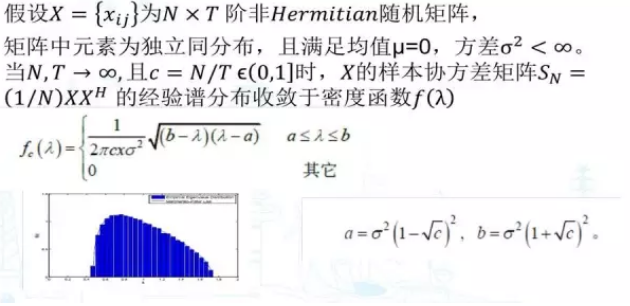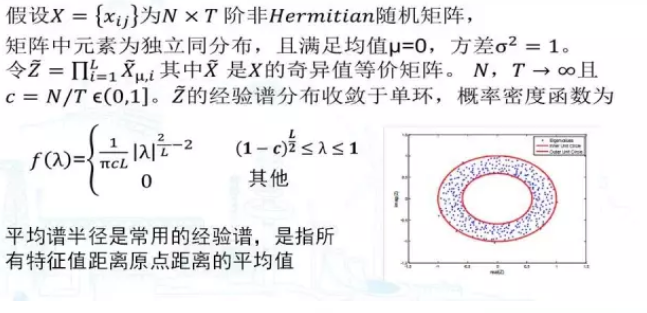##3 应用案例——静态稳定性

### 3.1 随机矩阵的构建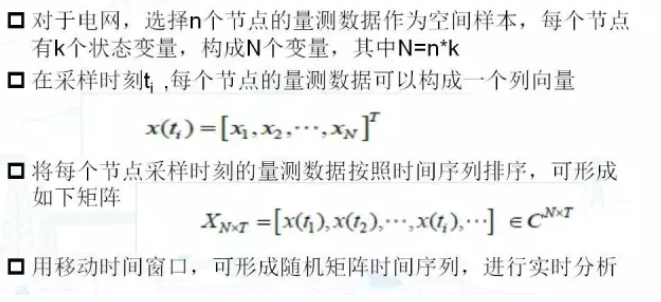### 3.2 应用于判定静态稳定性的方法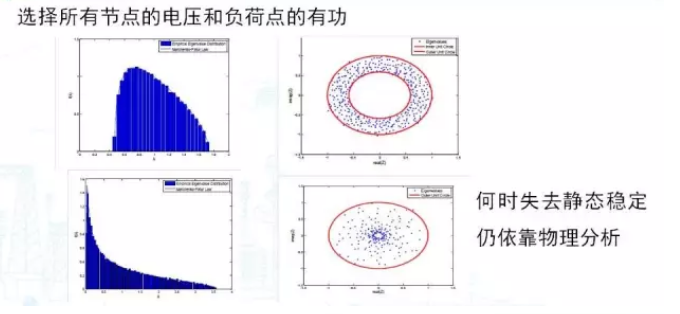###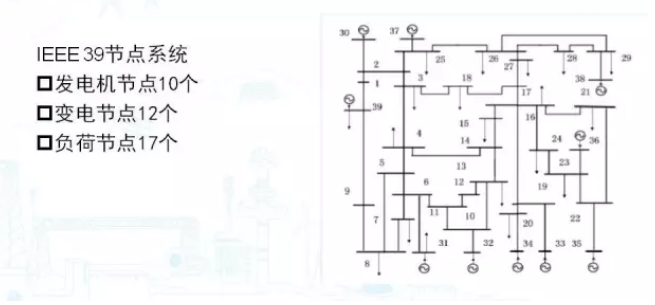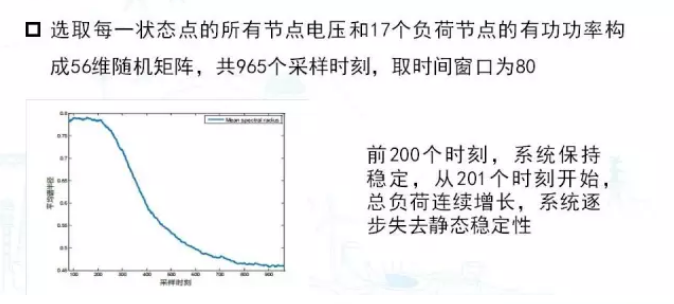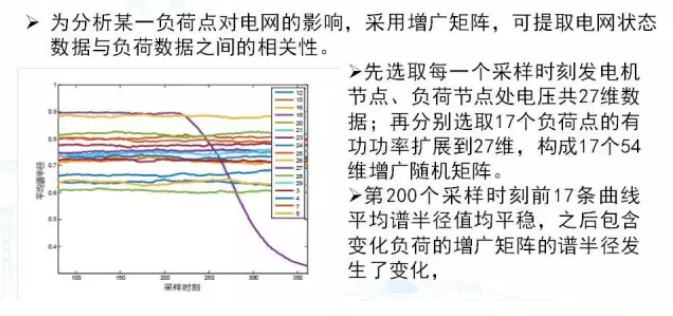## 4 应用案例——暂态稳定性

###展开全文• 在下面的代码中,我对一般的平方线性系统Ax=b实现了带有...我想通过求解Ax=b来测试我的实现,其中A是随机的100x100矩阵,b是随机的100x10向量。在我的代码中,我把矩阵A = np.array([[3.,2.,-4.],[2.,3.,3.],[5.,-3.,1...

在下面的代码中,我对一般的平方线性系统Ax=b实现了带有部分旋转的高斯消去。我测试了我的代码,它产生了正确的输出。不过,现在我正在尝试做以下事情,但我不太确定如何编码它,寻找一些帮助与此!

我想通过求解Ax=b来测试我的实现,其中A是随机的100x100矩阵,b是随机的100x10向量。

在我的代码中,我把矩阵

A = np.array([[3.,2.,-4.],[2.,3.,3.],[5.,-3.,1.]])

b = np.array([[3.],[15.],[14.]])

得到以下正确的输出:

[3. 1. 2.]

[3. 1. 2.]

但是现在我如何改变它来生成随机矩阵呢?

下面是我的代码:

import numpy as np

def GEPP(A, b, doPricing = True):

'''

Gaussian elimination with partial pivoting.

input: A is an n x n numpy matrix

b is an n x 1 numpy array

output: x is the solution of Ax=b

with the entries permuted in

accordance with the pivoting

done by the algorithm

post-condition: A and b have been modified.

'''

n = len(A)

if b.size != n:

raise ValueError("Invalid argument: incompatible sizes between"+

"A & b.", b.size, n)

# k represents the current pivot row. Since GE traverses the matrix in the

# upper right triangle, we also use k for indicating the k-th diagonal

# column index.

# Elimination

for k in range(n-1):

if doPricing:

# Pivot

maxindex = abs(A[k:,k]).argmax() + k

if A[maxindex, k] == 0:

raise ValueError("Matrix is singular.")

# Swap

if maxindex != k:

A[[k,maxindex]] = A[[maxindex, k]]

b[[k,maxindex]] = b[[maxindex, k]]

else:

if A[k, k] == 0:

raise ValueError("Pivot element is zero. Try setting doPricing to True.")

#Eliminate

for row in range(k+1, n):

multiplier = A[row,k]/A[k,k]

A[row, k:] = A[row, k:] - multiplier*A[k, k:]

b[row] = b[row] - multiplier*b[k]

# Back Substitution

x = np.zeros(n)

for k in range(n-1, -1, -1):

x[k] = (b[k] - np.dot(A[k,k+1:],x[k+1:]))/A[k,k]

return x

if __name__ == "__main__":

A = np.array([[3.,2.,-4.],[2.,3.,3.],[5.,-3.,1.]])

b = np.array([[3.],[15.],[14.]])

print (GEPP(np.copy(A), np.copy(b), doPricing = False))

print (GEPP(A,b))

展开全文• 生产随机矩阵的关键在于使用随机函数rand()。rand()表头文件:#include<stdlib.h>定义函数:intrand(void)函数说明:因为rand的内部实现是用线性同余法做的，他不是真的随机数，只不过是因为其周期特别长，所以...
• import numpy as npnp.random.rand(a, b)：>>> np.random.rand(4,3)array([[ 0.06679473, 0.71073515, 0.5694172 ],[ 0.95018143, 0.60161401, 0.8076899 ],[ 0.40341822, 0.72154255, 0.92283012],[ ...
• 你们知道Matlab如何生成随机矩阵吗?怎么样开启这项功能呢?对此感兴趣的用户可以随绿软吧去下文看看Matlab生成随机矩阵的详细方法。Matlab生成随机矩阵的详细方法首先需要知道Matlab中生成随机数的函数是rand，可以在...
• 1、随机矩阵 stochastic matrix 定义： 　A square matrix P is said to be stochastic if its elements are all nonnegative and all row sums are one. 　如果一个方阵P的每一项均为非负的并且每行的和均为1，则称...
• 生成随机数使用 random 模块 random.random() 用于随机生成一个0到1的浮点数 random.randint(start,stop) 随机生成[start,stop]区间内的整数 代码示例：import ...5))输出结果： 0.28113894170242715 2生成随机矩阵......